# Class 12 Mathematics: CBSE Sample Question Paper- Term I (2021-22)- 1 Notes | Study Mathematics (Maths) Class 12 - JEE

## JEE: Class 12 Mathematics: CBSE Sample Question Paper- Term I (2021-22)- 1 Notes | Study Mathematics (Maths) Class 12 - JEE

The document Class 12 Mathematics: CBSE Sample Question Paper- Term I (2021-22)- 1 Notes | Study Mathematics (Maths) Class 12 - JEE is a part of the JEE Course Mathematics (Maths) Class 12.
All you need of JEE at this link: JEE

Class-XII
Time: 90 Minutes
Max. Marks: 40

General Instructions :

1. This question paper contains three sections – A, B and C. Each part is compulsory.
2. Section - A has 20 MCQs, attempt any 16 out of 20.
3. Section - B has 20 MCQs, attempt any 16 out of 20.
4. Section - C has 10 MCQs, attempt any 8 out of 10.
5. There is no negative marking.
6. All questions carry equal marks

Section - A

Q.1: 1. What is the principal value branch of sec–1 x ?
(a) (–1, 1)
(b) [–1, 1]
(c)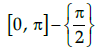(d) [0, π]

The sec function is periodic so to calculate its inverse function we need to make the function bijective. For that we have to consider an interval in which all values of the function exist and do not repeat. For sec function this interval is considered as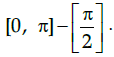Thus when we take the inverse of the function the domain becomes range and the range becomes domain. Hence the principal value branch is the range of sec–1x that isQ.2: What is the derivative of the function y = xtanx ?
(a) xtanx(xsecx + sec x logx)
(b)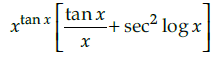(c) xtanx (2x sec x + tanx log x))
(d)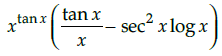y = xtan x
log y = tanx logx
Differentiating both side w.r.t. x.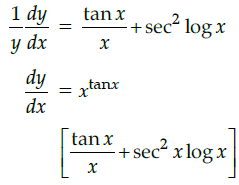Q.3: Matrix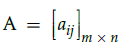is a square matrix if
(a) m > n
(b) m < n
(c) m = 1
(d) m = n

Given matrix is said to be square matrix if number of rows are equal to number of columns. Therefore,is a square matrix only if m = n.

Q.4: Calculate the determinant of the given matrix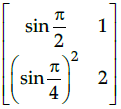(a) 1/2
(b) -1/2
(c) 3/2
(d) None of the above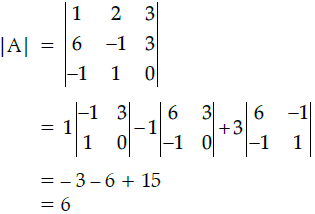Q.5: Absolute maximum of the function 2x + 5 in [5, 10] ?
(a) 5
(b) 10
(c) 20
(d) 25

Given, f '(x) = 2x + 5
Therefore, f '(x) = 2 > 0
Since, f '(x) > 0 in the maximum value is at upper and point f(10) = 2 × 10 + 5 = 25

Q.6: if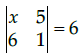then the value of x is:
(a) –6
(b) –36
(c) 6
(d) 36

For any matrices A and B of suitable orders, we have
(a) (A')' = A
(b) (A + B)' = A' + B'
(c) (kA)' = kA' (where k is any constant)
(d) (A B)' = B'A'

Q.7: Given set A = {a, b, c}. An identity relation in set A is:
(a) R= {(a, b), (a, c)}
(b) R= {(a, a), (b, b), (c, c)}
(c) R= {(a, a), (b, b), (c, c), (a, c)}
(d) R= {(c, a), (b, a), (a, a)}

Identity relation is function that always returns the same value that was used as its argument. That is, f(x) = x for all elements in set A.

Q.8: For a square matrix A = [aij] the quantity calculated for any element aij in A as the product of (-1)i+j and determinant of the square sub-matrix of order (n-1) obtained by leaving the ith row and jth column of A is known as
(a) Cofactor
(b) Minor
(c) Coefficient
(d) Elements

The cofactor of an element aij in A is calculated as the product of ( -1)i+j and determinant of the square sub-matrix of order (n-1) obtained by leaving the ith row and jth column of A.

Q.9: What is the absolute minimum of the function |x – 3| in the interval [4, 5] ?
(a) 2
(b) 4
(c) 6
(d) 8

Since the given function is increasing continuously in the given interval, maximum value is at the extreme end point.

Q.10: What is the general interval for sine function to become a bijective function?
(a)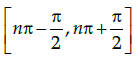(b)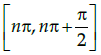(c)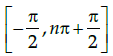(d)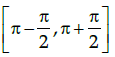The sine function is periodic so to calculate its inverse function we need to make the function bijective. For that we have to consider an interval in which all values of the function exist and do not repeat.

Q.11: Let R be relation from R to R the set of real numbers defined by R = {(x, y): x, y ∈ R and x – y + √3 is an irrational number}. Then, R is:
(a) Reflexive
(b) Transitive
(c) Symmetric
(d) An equivalence relation

For reflexive, let (x, x) ∈ R
⇒ x - x + √3 = √3 which is an irrational number. Hence, it is reflexive.
For symmetric, let f(x, y) ∈ R
⇒ x - y + √3 which is an irrational number.
This means y - x + √3 is an irrational number. So, f(y, x) ∈ R. Hence, it is symmetric.
For transitive, let f(x, y)∈ R
⇒ x - y + √3 and f(y, z) ∈ R
⇒ y - z + √3. Now adding these equations, we will get x - z + √3
⇒ (x, z)∈ R. Hence, it is transitive. Therefore, it is an equivalence relation.

Q.12: if x = at4, y = at3 then dy/dx will be
(a) 3/4t
(b) 3/4t2
(c) 3/4
(d) 3t/4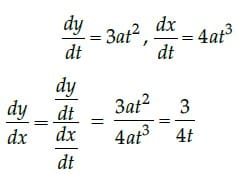Q.13: Every Identity matrix is a:

(a) Zero matrix
(b) Row matrix
(c) Scalar matrix
(d) Column matrix

A scalar matrix is an identity matrix when k = 1. But every identity matrix is clearly a scalar matrix.

Q.14: If y = sin x log x then the value of dy/dx is
(a) sin x log x – 1
(b)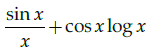(c)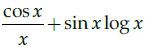(d)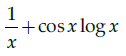y = sinx logx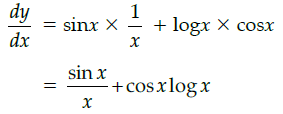Q.15: For Matrix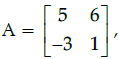(adj A)' is equal to
(a)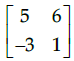(b)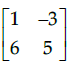(c)(d)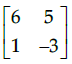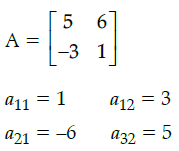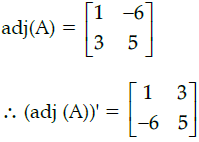Q.16: Which of the following line perpendicular to the tangent to curve y = x2 – 5 at x=1.
(a) 2y+x - 35 = 0
(b) 2x−3y+35 = 0
(c) 4x+7y+35 = 0
(d) 3x+7y+21= 0

Slope of tangent = dy/dx = 2x at x = 1, dy/dx = 2
slope of the perpendicular is - 1/slope
= - 1/2

Required equation is 2y + x – 35 =0

Q.17: Calculate the value of x such that the matrix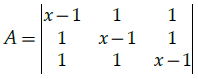is singular.
(a) –1, 2
(b) 2, 3
(c) 1
(d) No such value exist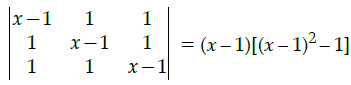+ 1[1 – x + 1] = 0

⇒ (x – 1)(x2 + 1 – 2x – 1) – x + 2 + 2 – x = 0

⇒ (x – 1)(x2 – 2x) – 2(x – 2) = 0

⇒ (x – 2)(x2 – x – 2) = 0

Q.18: If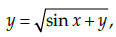then dy/dx is equal to
(a)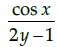(b)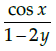(c)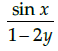(d)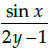Given that,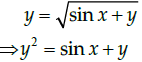Differentiate with respect to x, we have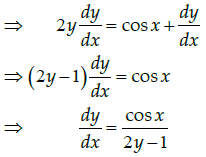Q.19: Maximize Z = x + y, subject to x – y ≤ –1, –x + y ≤ 0, x, y ≥ 0.
(a) the value of z is minimum at every point on line x – y = –1
(b) there is no feasible region with these constraints.
(c) the value of z is minimum at every point on line –x + y = 1
(d) None

The region determined by the constraints, is as follows. There is no feasible region and thus, Z has

x – y ≤ –1, –x + y ≤ 0, x, y≥ 0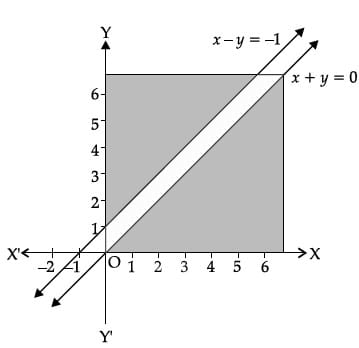Q.20: Which of the following is true for the given function?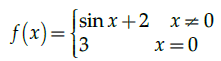(a) Continuous at x = 0
(b) Not continuous at 0
(c) differentiable at 0
(d) None of the above

Given,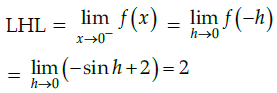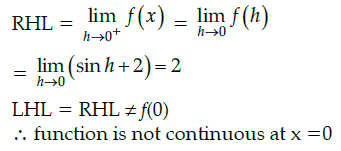Section - B

Q.21: Let A = {a, b, c} and B = {1, 2, 3} and f: A→ B is defined by f = {(a, 2), (b, 1), (c, 3)}. Is the function oneone and onto.
(a) both one-one and onto
(b) only one-one
(c) only onto
(d) neither of them

All the elements in the domain has a unique value in the range. Also the codomain of the function is equal to its range.

Q.22: Find the dy/dx of yx+xy = 0 ?
(a)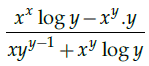(b)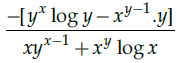(c) 0
(d) None of these

yx+xy = 0

or exlogy + eylogx = 0

Differentiating both sides w.r.t. x.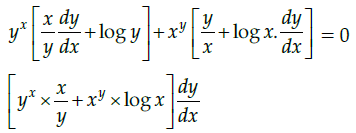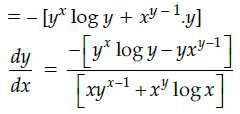Q.23: Corner points of the feasible region for an LPP are (0, 2), (3, 0), (6, 0), (6, 8) and (0, 5). Let F = 4x + 6y be the objective function.
The minimum value of F occurs at
(a) (0, 2) only
(b) (3, 0) only
(c) the mid-point of the line segment joining the points (0, 2) and (3, 0) only
(d) any point on the line segment joining the points (0, 2) and (3, 0)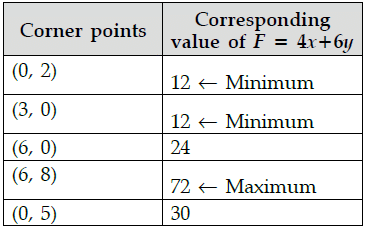Hence, minimum value of F occurs at any points on the line segment joining the points (0, 2) and (3, 0).

Q.24: Consider the curve y = x2/4. The Slope of the line parallel to tangent to the curve at x = 1 is
(a) 1/4
(b) 1/3
(c) - 1/2
(d) 1/2

y = x2/4
Slope of the curve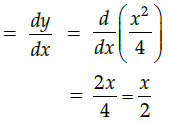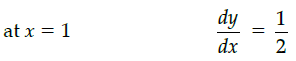Parallel lines have same slopes
∴ Slope of tangent = 1/2

Q.25: if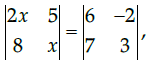then the value of x is
(a) 3
(b) ±3
(c) ±6
(d) 6

The process described in the reason statement is the correct procedure to solve the given question. For the given determinant,

2x2 – 40 = 32

2x2 = 72

x = ±6

Q.26: Given a function f (x)= 2x3 −21x2 +60x+48, it has local maximum at x =
(a) 2
(b) 3
(c) 5
(d) 4

f(x) = 2x3 – 21x2 + 60x + 48
f'(x) = 6x2 – 42x + 60
f'(x) = 0
⇒ 6x2 – 42x + 60 = 0
6 (x2 – 7x + 10) = 0
6 (x – 2) (x – 5) = 0
x = 2, 5

f''(x) = 12x – 42

f''(2) = –18 < 0

f''(5) = 60 – 42
= 18 > 0

∴ f(x) is maximum at x = 2.

Q.27: The principal value of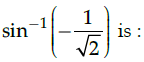(a) π/4
(b) π/6
(c) -π/4
(d) π/3

The principal value of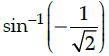means that we need to find an angle in the principal branch of the function where the sine function is equal to - 1/√2. Hence the required value is -π/4.

Q.28: Suppose P and Q are two different matrices of order 3 × n and n × p, then the order of the matrix P × Q is ?
(a) 3 × p
(b) p × 3
(c) n × n
(d) 3 × 3

Q.29: Let f(x) = |sin x|, then
(a) f is everywhere differentiable
(b) f is everywhere continuous but not differentiable at x = n π, n ∈ Z.
(c) f is everywhere continuous but not differentiable at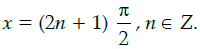(d) none of these

Given that, f(x) = |sin x|
The functions |x| and sin x are continuous function for all real value of x.
Thus, the function f(x) = |sin x| is continuous function everywhere.
Now, |x| is non-differentiable function at x = 0.
Since f(x) = |sin x| is non-differentiable function at sin x = 0
Thus, f is everywhere continuous but not differentiable at x = n π, n ∈ Z.

Q.30: If function f : R → R defined as f(x) = x2 then f(x) is
(a) onto
(b) one-one and onto
(c) one-one
(d) None of these

f(x) is a one-one function
if f(x1) = f(x2) ⇒ x1= x2
Let f(x1) = f(x2) for some x1, x2 ∈ R
⇒(x1)2=(x2)2
⇒ x1 = ±x2
Hence f(x) is one-one.

Q.31: Which of these intervals, the function f (x)= √2 cos x+x−35 is monotonic?
(a)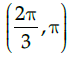(b)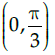(c)(d)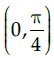A function is Monotonic if its first derivative’s sign doesn’t change in the given interval.

Q.32: If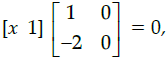then x equals
(a) 0
(b) -2
(c) -1
(d) 2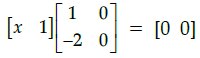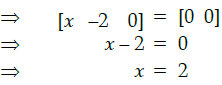Q.33: Objective function:
Maximise Z = 1000x + 600y
Constraints:
x + y ≥ 200
y ≥ 20, x ≥ 0
4x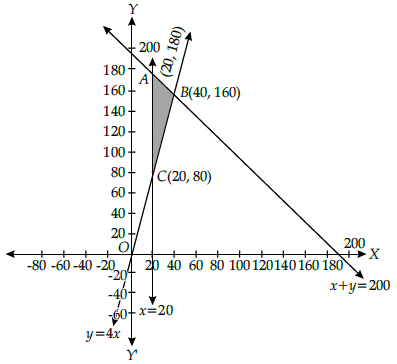Z is maximum at point
(a) (20, 80)
(b) (20, 180)
(c) (0, 0)
(d) (40, 160)

The corner points are A(20, 180), B(40, 160), C(20, 80)

Evaluating the objective function Z = 1,000x + 600y at A, B and C
At A(20, 180),
Z = 1,000 × 20 + 600 × 180 = 20,000 + 1,08,000 = ₹1,28,000
At B(40, 160), Z = 1,000 × 40 + 600 × 160 = 40,000 + 96,000 = ₹1,36,000 (max.)
At C(20, 80), Z = 1000 × 20 + 600 × 80 = 20,000 + 48,000 = ₹68,000 or
Z is maximum, when x = 40, y = 160.

Q.34: A particle moves along the curve x2 = 2y. The point at which, ordinate increases at the same rate as the abscissa is ________
(a) (1,2)
(b) (1/2, 1)
(c) (1/2, 1/2)
(d) (1, 1/2)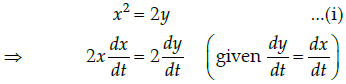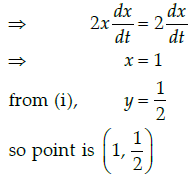Q.35: If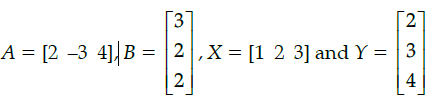then AB + XY equals
(a) 
(b) 
(c) 28
(d) 24

Given, A = [2 -3 4] ,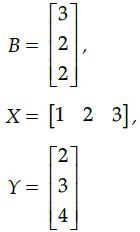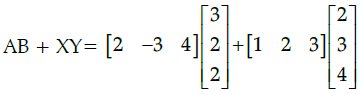= [6 – 6 + 8] + [2 + 6 + 12]

=  + 

= 

Q.36: If function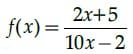Then the domain of the function is:
(a)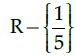(b) R
(c) R - {1}
(d) R - {5}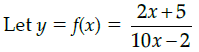10xy – 2y = 2x + 5
10xy – 2x = 5 + 2y

2x(5y – 1) = 5 + 2y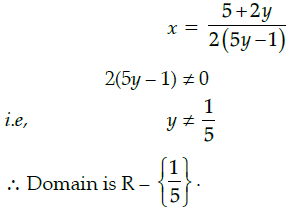Q.37: The maximum number of equivalence relations on the set A = {1, 2, 3} are
(a) 1
(b) 2
(c) 3
(d) 5

Given that, A = {1, 2, 3}
Now, number of equivalence relations are as follows:
R1 = {(1, 1), (2, 2), (3, 3)}
R2 = {(1, 1), (2, 2), (3, 3), (1, 2), (2, 1)}
R3 = {(1, 1), (2, 2), (3, 3), (1, 3), (3, 1)}
R4 = {(1, 1), (2, 2), (3, 3), (2, 3), (3, 2)}
R5 = {(1, 2, 3) ⇔ A × A = A2}
∴ Maximum number of equivalence relations on the set A = {1, 2, 3} = 5

Q.38: If A is any square matrix of order 3 × 3 such that |A| = 3, then the value of |adj A| is?
(a) 3
(b) 1/3
(c) 9
(d) 27

|A| = 3, n = 3

|adj A| = |A|2 = 32 = 9

Q.39: The equation of tangent to the curve y(1 + x2) = 2 – x, where it crosses x-axis is:
(a) x + 5y = 2
(b) x – 5y = 2
(c) 5x – y = 2
(d) 5x + y = 2

Given that the equation of curve is
y(1 + x2) = 2 – x ...(i)
On differentiating with respect to x, we get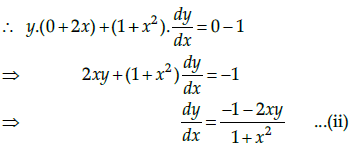Since, the given curve passes through -axis,
i.e    y = 0
∴  0(1 + x2) = 2 - x   [by using eq. (i) ]
⇒ x = 2
So the curve passes through the point (2, 0).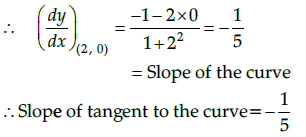∴ Equation of tangent to the curve passsing through (2, 0) is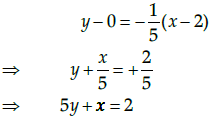Q.40: A = [aij]m×n is a square matrix, if
(a) m < n
(b) m > n
(c) m = n
(d) None of these

It is known that a given matrix is said to be a square matrix if the number of rows is equal to the number of columns.
Therefore,
A = [aij]m x n is a square matrix, if m = n.

Section - C

Q.41: For an objective function Z = ax + by, where a, b > 0; the corner points of the feasible region determined by a set of constraints (linear inequalities) are (0, 20), (10, 10), (30, 30) and (0, 40). The condition on a and b such that the maximum Z occurs at both the points (30, 30) and (0, 40) is:
(a) b − 3a = 0
(b) a = 3b
(c) a + 2b = 0
(d) 2a − b = 0

As Z is maximum at (30, 30) and (0, 40)

⇒ 30a + b = 40b

⇒ b – 3a = 0

Q.42: For which value of m is the line y = mx + 1 a tangent to the curve y2 = 4x?
(a) 1 2
(b) 1
(c) 2
(d) 3

y = mx + 1 ...(i)

and y2 = 4x ...(ii)
Substituting (i) in (ii) :

(mx + 1)2 = 4x

⇒ m2x2 + (2m – 4)x + 1 = 0 ...(iii)
As line is tangent to the curve

⇒ line touches the curve at only one point

⇒ (2m – 4)2 – 4m2 = 0

⇒ m = 1

Q.43: The maximum value of [x(x+1)+1]1/3, 0 ≤ x ≤ 1 is:
(a) 0
(b) 1/2
(c) 1
(d)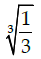Let f(x) = [x(x – 1) + 1]1/3,0 ≤ x ≤ 1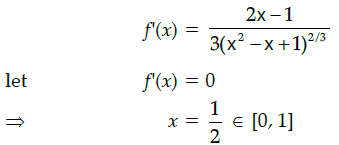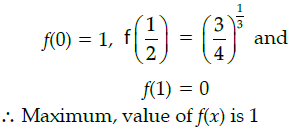Q.44: In a linear programming problem, the constraints on the decision variables x and y are x − 3y ≥ 0, y ≥ 0, 0 ≤ x ≤ 3. The feasible region
(a) is not in the first quadrant
(b) is bounded in the first quadrant
(c) is unbounded in the first quadrant
(d) does not exist

Feasible region is bounded in the first quadrant

Q.45: Let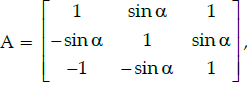where 0 ≤ α ≤ 2π, then:
(A) |A|= 0
(B) |A| ∈ (2, ∞)
(C) |A| ∈ (2, 4)
(D) |A| ∈ [2, 4]

|A| = 2 + 2sin2θ

As –1 ≤ sin θ ≤ 1, ∀ 0 ≤ θ ≤ 2π

⇒ 2 ≤ 2 + 2sin2θ ≤ 4

⇒ |A|∈ [2, 4]

Questions 46-50 are based on a Case-Study

Case-Study

The fuel cost per hour for running a train is proportional to the square of the speed it generates in km per hour. If the fuel costs ₹48 per hour at speed 16 km per hour and the fixed charges to run the train amount to ₹1200 per hour. Assume the speed of the train as v km/h. Based on the given information, answer the following questions.Q.46: Given that the fuel cost per hour is k times the square of the speed the train generates in km/h, the value of k is:
(a) 16/3
(b) 1/3
(c) 3
(d) 3/16

Fuel cost = k(speed)2

⇒ 48 = k.162
⇒ k = 3/16

Q.47: If the train has travelled a distance of 500km, then the total cost of running the train is given by function:
(a)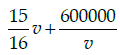(b)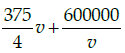(c)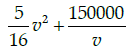(d)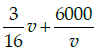Total cost of running train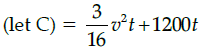Distance covered = 500 km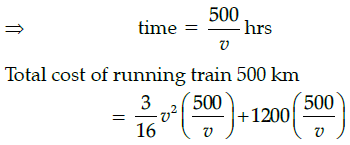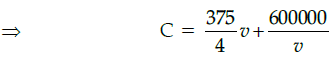Q.48: The most economical speed to run the train is:
(a) 18km/h
(b) 5km/h
(c) 80km/h
(d) 40km/h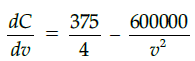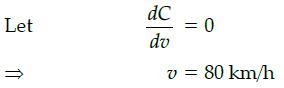Q.49: The fuel cost for the train to travel 500 km at the most economical speed is: (a) ₹3750
(b) 750
(c) 7500
(d) 75000

Fuel cost for running 500 km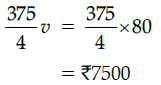Q.50: The total cost of the train to travel 500km at the most economical speed is:
(a) 3750
(b) 75000
(c) 7500
(d) 15000

Total cost for running 500 km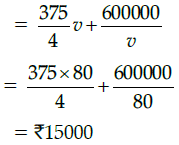The document Class 12 Mathematics: CBSE Sample Question Paper- Term I (2021-22)- 1 Notes | Study Mathematics (Maths) Class 12 - JEE is a part of the JEE Course Mathematics (Maths) Class 12.
All you need of JEE at this link: JEEUse Code STAYHOME200 and get INR 200 additional OFF

## Mathematics (Maths) Class 12

209 videos|209 docs|139 tests

Track your progress, build streaks, highlight & save important lessons and more!

,

,

,

,

,

,

,

,

,

,

,

,

,

,

,

,

,

,

,

,

,

;